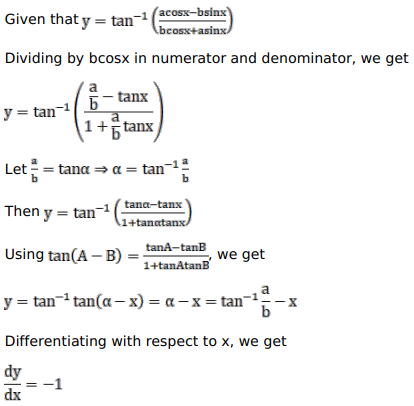# Solve this followingQuestion:

Mark $(\sqrt{)})$ against the correct answer in the following:

If $y=\tan ^{-1}\left(\frac{a \cos x-b \sin x}{b \cos x+a \sin x}\right)$ then $\frac{d y}{d x}=?$

A. $\frac{a}{b}$

B. $\frac{-b}{a}$

C. 1

D. $-1$

Solution: